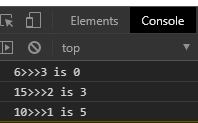# JavaScript Unsigned Right Shift Assignment Operator

In JavaScript “>>>=” is known as the unsigned right shift assignment bitwise operator. This operator is used to move the particular amount of bits to the right and returns a number that is assigned to a variable.

Syntax:

```a >>>= b

Meaning: a = a >>> b```

Return value: It returns the number after shifting of bits.

Example 1:

## HTML

 ` ` `<``html` `lang``=``"en"``> ` ` `  `<``head``> ` `    ``<``meta` `charset``=``"UTF-8"``> ` `    ``<``meta` `name``=``"viewport"` `content``= ` `        ``"width=device-width, initial-scale=1.0"``> ` ` ` ` `  `<``body``> ` `    ``<``script``> ` `        ``let a = 16 ` `        ``let b = 2 ` `        ``console.log(`\${a}>>>=\${b} is \${a >>>= b}`) ` `    `` ` ` ` ` `  ` `

Output:Example 2: Using a variable to store the return value by >>> operator.

## HTML

 ` ` `<``html` `lang``=``"en"``> ` ` `  `<``head``> ` `    ``<``meta` `charset``=``"UTF-8"``> ` `    ``<``meta` `name``=``"viewport"` `content``= ` `        ``"width=device-width, initial-scale=1.0"``> ` ` ` ` `  `<``body``> ` `    ``<``script``> ` `        ``let a = 6 ` `        ``let b = 3 ` `        ``c = a >>> b ` `        ``console.log(`\${a}>>>\${b} is \${c}`) ` `        ``console.log(`\${15}>>>\${2} is \${15 >>> 2}`) ` `        ``console.log(`\${10}>>>\${1} is \${10 >>> 1}`) ` `    `` ` ` ` ` `  ` `

Output:My Personal Notes arrow_drop_upCheck out this Author's contributed articles.

If you like GeeksforGeeks and would like to contribute, you can also write an article using contribute.geeksforgeeks.org or mail your article to contribute@geeksforgeeks.org. See your article appearing on the GeeksforGeeks main page and help other Geeks.

Please Improve this article if you find anything incorrect by clicking on the "Improve Article" button below.

Article Tags :
Practice Tags :

Be the First to upvote.

Please write to us at contribute@geeksforgeeks.org to report any issue with the above content.